# Calculating The Covariance Matrix And Portfolio Variance

In this blog, we will learn how to create the covariance matrix for a portfolio of n stocks for a period of ‘m’ days.

The covariance matrix is used to calculate the standard deviation of a portfolio of stocks which in turn is used by portfolio managers to quantify the risk associated with a particular portfolio.

## How Does Portfolio Analysis Work?

Let us understand how portfolio analysis works. Say we have 4 stocks in our portfolio and we want to allocate optimal capital to each of these stocks, such that our risk is minimum. To do this, we need to first create multiple portfolios with different weights reflecting different capital allocations to each stock and calculate the standard deviation of each of the resulting portfolios and then choose the one with the lowest risk.

Expected portfolio variance= SQRT (WT * (Covariance Matrix) * W)

The above equation gives us the standard deviation of a portfolio, in other words, the risk associated with a portfolio. In this equation, 'W' is the weights that signify the capital allocation and the covariance matrix signifies the interdependence of each stock on the other. 'WT' is the transpose of the same weights matrix.Let us understand what Covariance is and how to calculate it for multiple stocks.

## What Is Covariance?

Covariance is a measure of the joint variability of two random variables.

• If the two variables increase and decrease simultaneously then the covariance value will be positive.
• Conversely if one increases while the other decreases then the covariance will be negative.

## How To Calculate Covariance?

Let us understand in a stepwise manner how to calculate the covariance for 'n' different stocks in the portfolio.

Let us say that the ‘n’ stocks in our portfolio (S1,S2,…Sn) have closed price as given below

### 1. Stock DataWe will combine this stock data in a single matrix and name it as 'S':### 2. Average Price Of Stock

As you can see each stock consists of the past ‘m’ days close prices. Using this data, we will first compute the average price for each stock.

For example, the mean price for stock 'S1' is given as follows: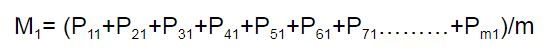Next, we save all the means of 'n' stocks in a matrix called 'M' as follows: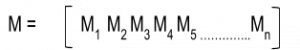Our ultimate aim is to understand how one stock’s behaviour is related to that of another’s. To compare two stocks with two completely different price ranges, we need to first establish a common base. So to make the comparison of stocks movements even, we subtract the mean of the stock price from the stock price.

This will create a new de-meaned stock price which will help in comparing how one stock's movement from its mean is dependent on another’s movement from it’s mean. Let us understand how to create a de-meaned series.

### 3. Demeaning The Prices

First, we subtract the mean stock price from the close prices of the corresponding stock. This is will give us the matrix with de-meaned scores, or a measure of how far a data point is from its mean.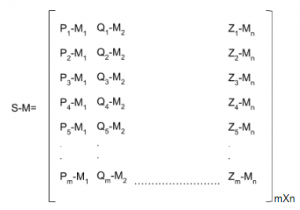### 4. Covariance Matrix

Once we have the de-meaned price series, we establish the covariance of different stocks by multiplying the transpose of the de-meaned price series with itself and divide it by 'm' (number of data points), this gives us the covariance matrix: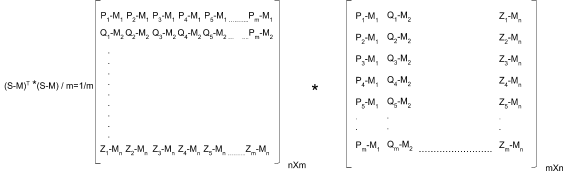In the resulting covariance matrix, the diagonal elements represent the variance of the stocks.

Also, the covariance matrix is symmetric along the diagonal, meaning:

σ21 = σ12

### 5. Portfolio Variance

Once we have the covariance of all the stocks in the portfolio, we need to calculate the standard deviation of the portfolio. To do this, we first need to decide the weights or percentage capital allocation for each stock.

While creating the weights matrix we need to keep in mind that the sum of all individual components in the matrix should be equal to 1, since they are a percentage of the total capital invested.

For a portfolio containing 'n' stocks, and a weight distribution given by the matrix 'W'The portfolio's expected returns is given by:

Expected portfolio return = M * W

The portfolio’s variance is given by

Expected portfolio variance= WT * (Covariance Matrix) * W

Once we have calculated the portfolio variance, we can calculate the standard deviation or volatility of the portfolio by taking the square root the variance. One can construct various portfolios by changing the capital allocation weights the stocks in the portfolio. Once you plot these portfolios in a graph it would look something like this: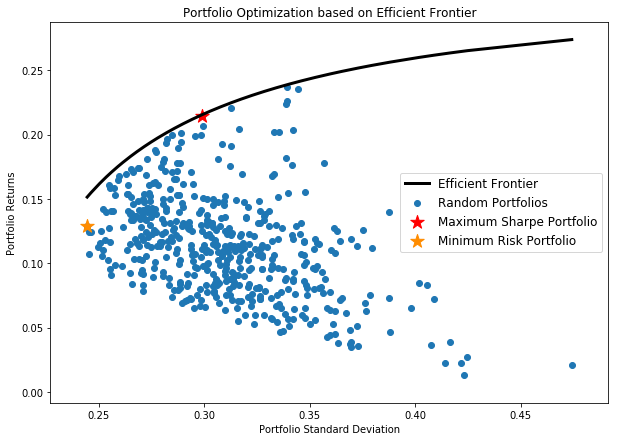Here the black line indicates the efficient frontier which signifies the maximum profit one can expect for a given risk. Using this plot, you can easily obtain the portfolio weight combinations for different requirements, such as least risk and highest Sharpe ratio. Say you are a pension fund manager trying to minimize the risk you would choose a portfolio highlighted by the yellow star.

Similarly, if you are hedge fund manager trying for the best risk-reward combination, you would choose a set of portfolio weights highlighted by the red star.

You can learn how to use MPT (Modern Portfolio Theory) and other techniques such as Style Investing from our course on Quantitative Portfolio Management.